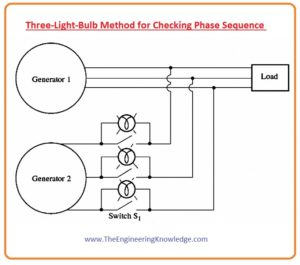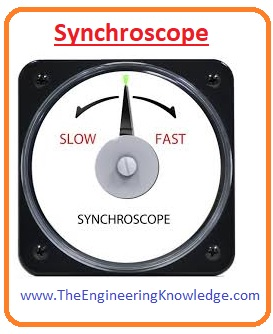Hello friends, I hope all of you are fine. In today’s tutorial, we are gonna have a look at the Parallel Operation of Synchronous Generator. Nowadays it is rare in an electrical system that any synchronous generator providing power to its loads is lonely. In certain conditions, like if somewhere supply is disconnected from the grid, then there is a single generator can be used to provide power to the load, but its normal generator does not work alone. In normal working conditions always more than one generator is working together in parallel. An example of this system is the united states of America grid station, where almost a thousand plus generators provide power to the load in the system.

In today’s post, we will have a look at how a generator works in parallel, its features, connections, and some other conditions. So, let’s get started with a Parallel Operation of Synchronous Generator.

## Parallel Operation of Synchronous Generator

• Before study the parallel operation of the generators, first we discuss the advantage of the parallel operation. That are described here.

## Conditions for Parallel Operation of Synchronous Generator

• In above-given diagram, you can see that there are two generators connected in parallel. The first generator is G1 provides electrical power to output with the second generator G2, which is connected with the first by a switch S
• If we closed the switch randomly at any time and connect these generators it can cause of serious disaster for both the generator and load.
• And the voltage at the terminals of both generators is not the same, it will produce such a high current that will damage the generators.
• To eliminate this situation there should be all three phases of both generators should be at the same voltage level and have the same phase angles.
• It means phase a has an equal voltage level to phase a’, phase b is equal to phase b’ and c is equal to c’.
• To get this same matching of the phases we must follow some conditions that are described here.
1. The root mean square (rms) voltage of these 2 generators should be the same.
2. The phase sequence of both of the generators should be the same, which means a is connected with a’ of the other generator, b with b’, and c with c’.
3. The phase angle of two similar phases should be equal. This means (a) the phase of both the generators have the same angle, and so on.
4. The frequency of the generator that is going to connect also called the ongoing generator should be a little bit higher than the generator that is already in working condition.
• Now we explain these 4 conditions in detail.

Condition 1 and 3:

• To have similar voltage at the terminals of both generators there should be the same value of root mean square voltage and their phase angle that are described in condition 3. The voltage at phases a and a’ will remains the same during working if these to follow conditions one and three.

Condition 2:

The phases that are to be connected have a similar phase sequence.

Condition 4:

• If the frequency of the ongoing generator is not slightly higher than that of running the generator, there will a huge amount of transient’s current will produce until the frequencies of both generators become equal.
• The frequencies of these 2 generators should be almost equal, but not exactly the same value, which means the ongoing generator frequency should be a little bit higher than another generator.
• There should be some difference among the frequencies of 2 generators so that the incoming generator phase angle will change according to the phase angle of the running generator.
• By this method, the angles among the voltage can be observed and the S1 can be closed when both generators have same value of the voltages.

## Procedure for Paralleling Generators

### Three-Light-Bulb Method for Checking Phase Sequence

• There is another method to check the phase sequence of the generator.
• In this method, there are 3 bulbs connected with the open terminals of the incoming generator with the switches that connect it with the system. it is shown in a given diagram.• If there is a difference among the sequence of the phases, then the bulb glows (it means high phase difference) and then becomes darker (less phase difference).
• If these 3 bulbs glow and dim at the same time, then both of the generators is connected with the same phases.
• If the bulbs do not glow at a time or glow one by one then there will be different phases that are connected, to solve this problem one of the sequences should be changed.
• The other point for parallel operation is that the frequency of the incoming generator should a little bit higher than the working generator.
• The value of the frequency is set by the frequency meter, then measure from the phase changing (difference).
• The frequency of the coming generator should be slightly higher than the system so that when it is connected with the system it works as a generator, not as a motor.
• When the frequencies of both the working and incoming generators are almost the same, the 2 generators will vary phase according to each other gradually,
• The variations in the phase detect, and when the angles of the phases are the same, the switch that joins both the generator will close.

## How can we tell when the 2 generators are in phase?

• The simplest method is to watch the three bulbs that are connected with the circuitry as shown in the above figure.
• When all three bulbs glow at a time there is no phase difference and phases are connected in the same sequence.
• This method works but has some limitations, sometimes not working accurately.
• There is another technique that can be used for checking the phase sequence, is the use of
• It is an instrument that calculates the phase angle difference among (a) phases of the generators.
• In the given diagram, you can see the synchroscope.• You can see the needle on the meter that will indicate the phases if it is at zero and a one-eighty degree then the voltages are in phases.
• As we studied earlier that the frequencies of both generators are different, so the angle of the phases will vary on the meter gradually.
• If the frequency of the incoming generator is higher than the working generator (that is in our case), the angle of the phase will advance and the pointer (needle) on the meter will move in the clockwise direction.
• If the frequency of the incoming generator is less than the running generator, the needle of the meter will move in the anticlockwise direction.
• When the needle of the meter is either 0 degrees or 1800 it means that the phase sequence is the same, so we can close the switch to make contact among the generators.
• You can note that this meter tells about the only single phase, that we discussed. It does not tell about the sequences of the phases.
• For high-rating generators that are connected to a power system, parallel operation of the incoming generator is done by the computer, a synchroscope is not used for such systems.

## Advantages of Parallel Operation of Generators or Alternators

1. Increased Power Output: Though operating the generators in parallel conditions the total power of the system is increased. It is good for conditions where one generator is not able to fulfill the load’s demand. The parallel operation of generation helps many generators to share loads, by providing high total power capacity.
2. Redundancy and Reliability: Parallel operation offers redundancy if generators stop working. If one generator is damaged or not working other generators continue to provide power to load without any interruption. It will increase the reliability of the system
3. Load Sharing: Parallel operation helps to share loads in generators. Every generator provides share some part of the load and the load is uniformly distributed in generators on the base of capacity. it avoids overloading of one generator and provides effective working of the system
4. Flexibility and Scalability: Parallel operation helps to provide flexibility and scalability in the power production system. Extra generators can easily add parallel configurations to fulfill the load’s demands. Generators for maintenance can e removed without disturbing the supply to load
5. Improved Efficiency: This working configuration of generators causes good fuel efficiency. When load needs is low it is more effective to work less number of the generator at high larger loads than operating one generator at a low load.
6. Voltage Regulation: Through this operation, good voltage regulation is obtained. Multiplegernatos help stable the voltage values in the system, by providing voltage variations and making sure constant power supply
7. Fault Tolerance: Through this process, we can increase the fault tolerance of the system. If there is a fault exists in one generator another can compensate and provide a supply to load. It will reduce the faculty impacts and make sure regular operation

## Precautions During Parallel Connection

• The voltage of all generators must be equal. If the voltage value is not small there will be a circulating current between generators that can damage the generator or devices connected.
• The phase angle of all generators must be equal. If the phase angle is not the same there will be torque imbalance between senators that causes generators to Overspeed
• The frequency of all generators must be equal. Due to frequency unequal condition, there is a slip between generators that can cause generators to overheat and damage devices connected
• The polarity of all generators must be the same. A short circuit occurs if polarities are not the same
• The load on all generators must be balanced. Imbalance load causes circulating current in generators that damage the generators.
• The generators must be synchronized before they are connected in parallel. Synchronization makes sure that generators are operating the same freqeuncy and phase angle before connected in the system

You can also read some related topics to synchronous generators that are listed here.

Introduction to Synchronous Generator

Synchronous Generator Equivalent Circuit

Synchronous Generator Phasor Diagram

Synchronous Generator Power and Torque

Synchronous Generator Parameters

Synchronous Generator Operating Alone

Synchronous Generator parallel with Large Power system

Synchronous Generator Parallel with same Size Generator

Synchronous Generator Ratings

Synchronous Generator Capability Curves

Synchronous Generator Transients

That is a complete article on the Parallel Operation of Synchronous Generator if you want to know something more can ask in the comments. See you in the next tutorial Synchronous Generators in Parallel with Large Power Systems.